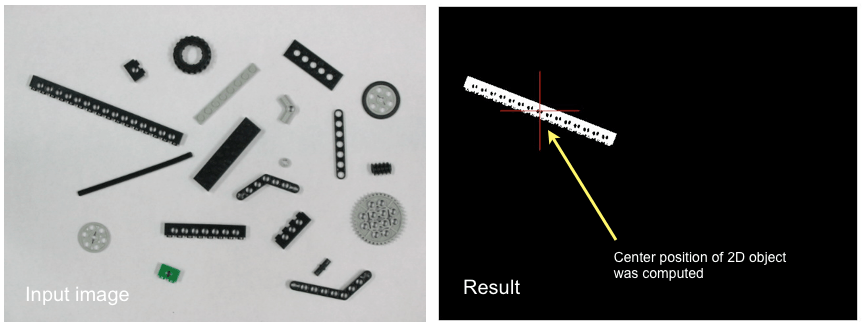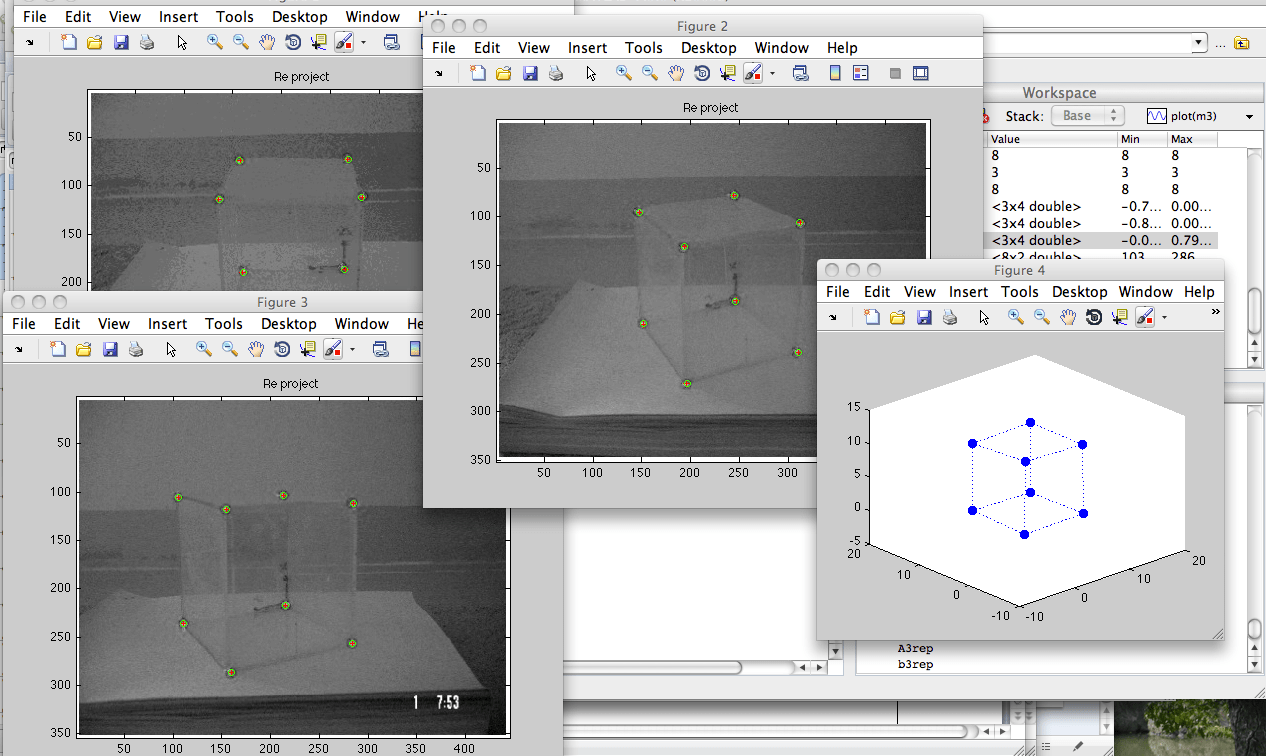1. Computer vision tutorial (research log).

2. Matlab code: center of mass algoritm

This algorithm is useful to compute center of mass using moment of 2D object. Real-time implementation of this algorithm is useful to perform 2D object tracking (for example: tracking a moving car, computing position of pupil in eye tracking, etc.)sdsds

2. Matlab code: 3D reconstruction from multiple images based on user’s input

This algorithm is useful to create a basic 3D model from multiple images. The camera matrix and 3D model are computed based on user’s input. This source code is useful to perform camera calibration using solid 3D object as well as to understand basic principle of 3D reconstruction algorithm.3. Matlab code: simulation of 3D gaze tracking algorithm